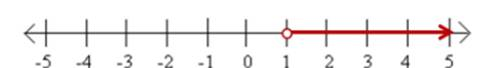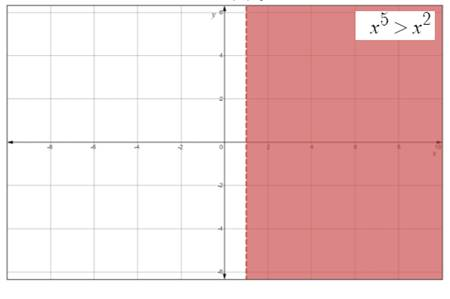# the nonlinear inequality. Express the solution using interval notation and graph the solution set.### Precalculus: Mathematics for Calcu...

6th Edition
Stewart + 5 others
Publisher: Cengage Learning
ISBN: 9780840068071### Precalculus: Mathematics for Calcu...

6th Edition
Stewart + 5 others
Publisher: Cengage Learning
ISBN: 9780840068071

#### Solutions

Chapter 1.7, Problem 72E
To determine

## To solve: the nonlinear inequality. Express the solution using interval notation and graph the solution set.

Expert Solution

x(1,) .

### Explanation of Solution

Given:

The given inequality is x5>x2 .

Concept used:

Guidelines for solving nonlinear inequality:

1. Move all terms to one side.
2. Factor the non-zero side of the inequality.
3. Find the value for which each factor is zero. The number will divide the real lines into interval. List the interval determined by these numbers.
4. Make a table or diagram by using test values of the signs of each factor on each interval. In the last row of the table determining the sign of the product of these factors.
5. Determine the solution of the inequality from the last row of the sign table.

Calculation:

The given inequality can be expressed as

x5>x2{given}x3>1{divide by x2}x>1{simplify} .

Hence,the solution set is x(1,) .

The solution set of the inequality graphed on the number line.The graph of the non-linear inequality x5>x2 is:### Have a homework question?

Subscribe to bartleby learn! Ask subject matter experts 30 homework questions each month. Plus, you’ll have access to millions of step-by-step textbook answers!